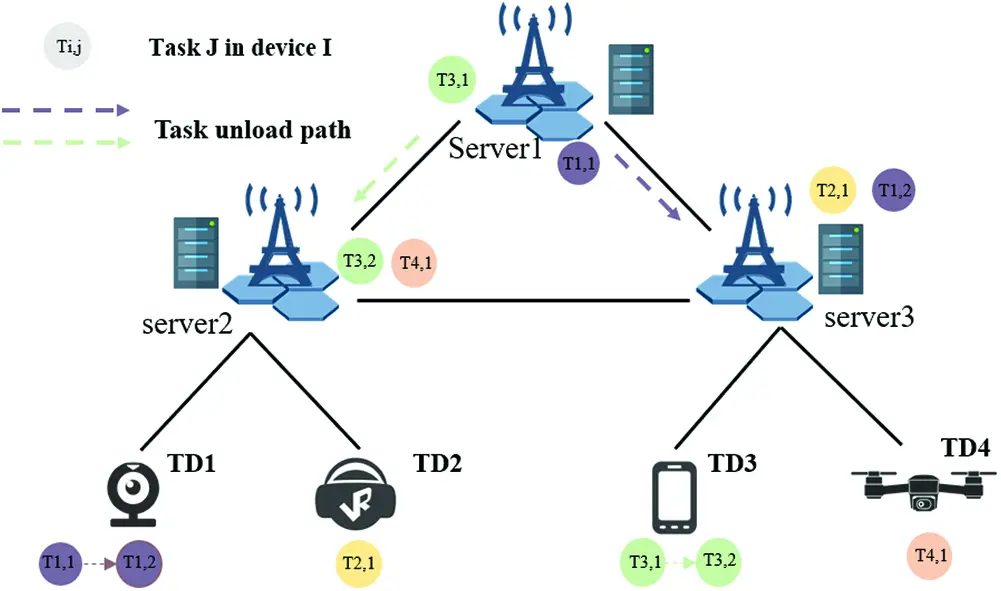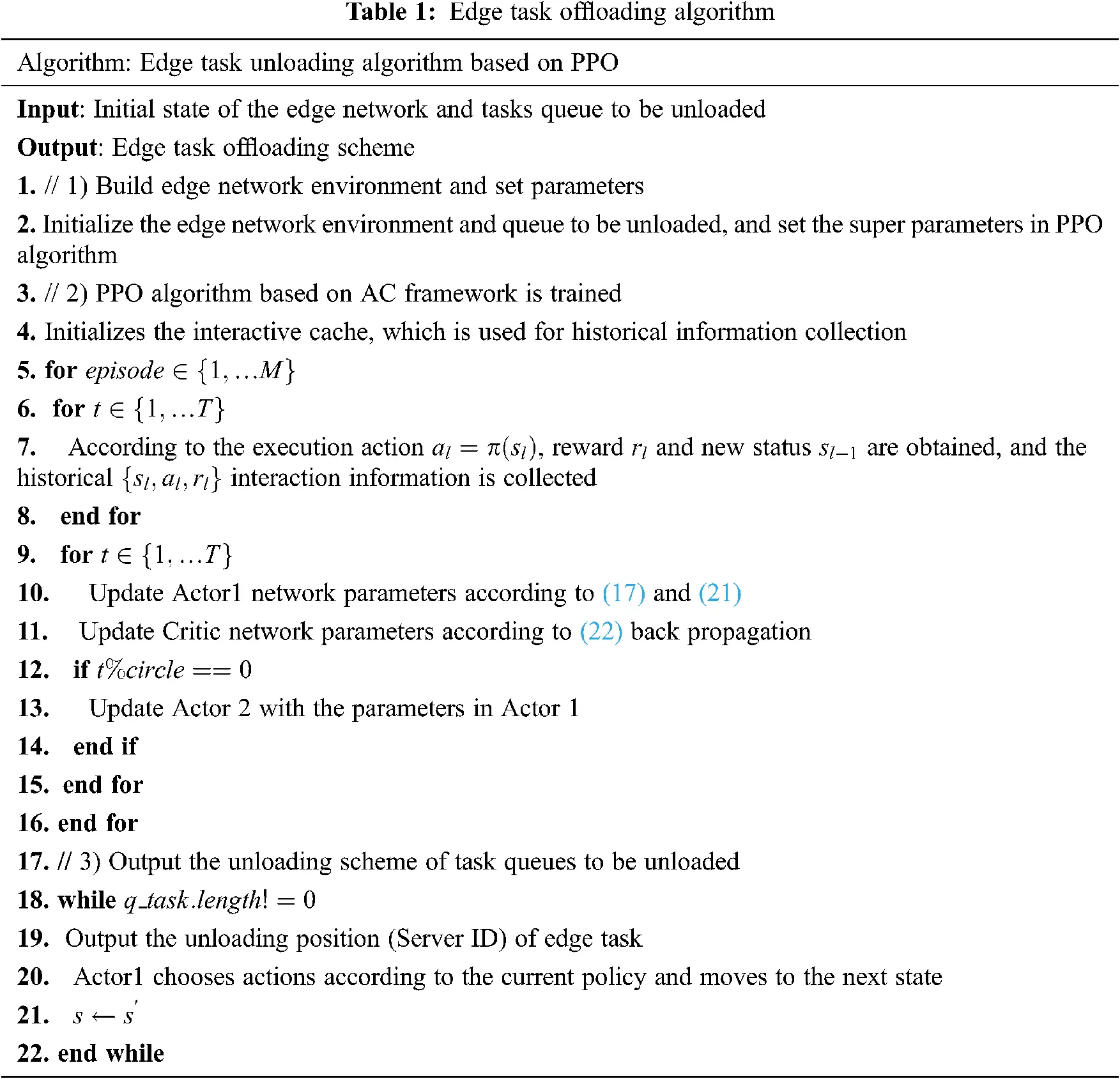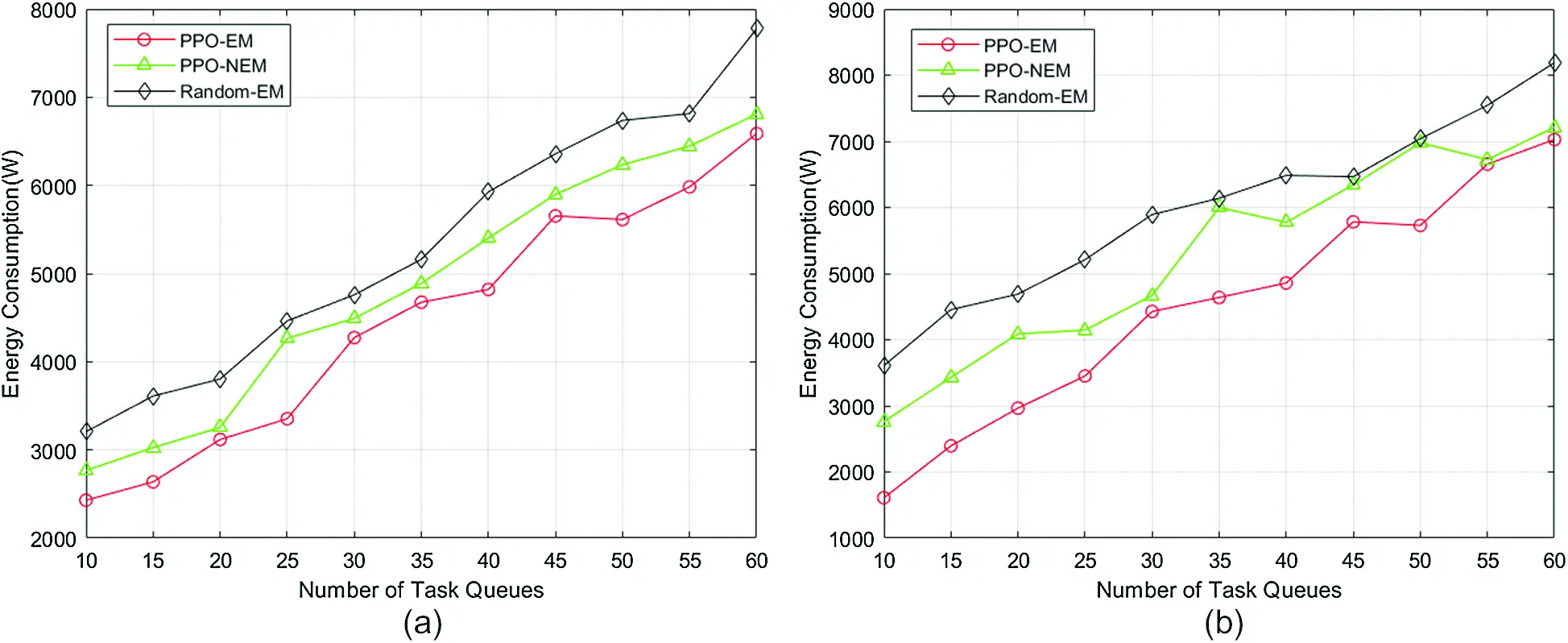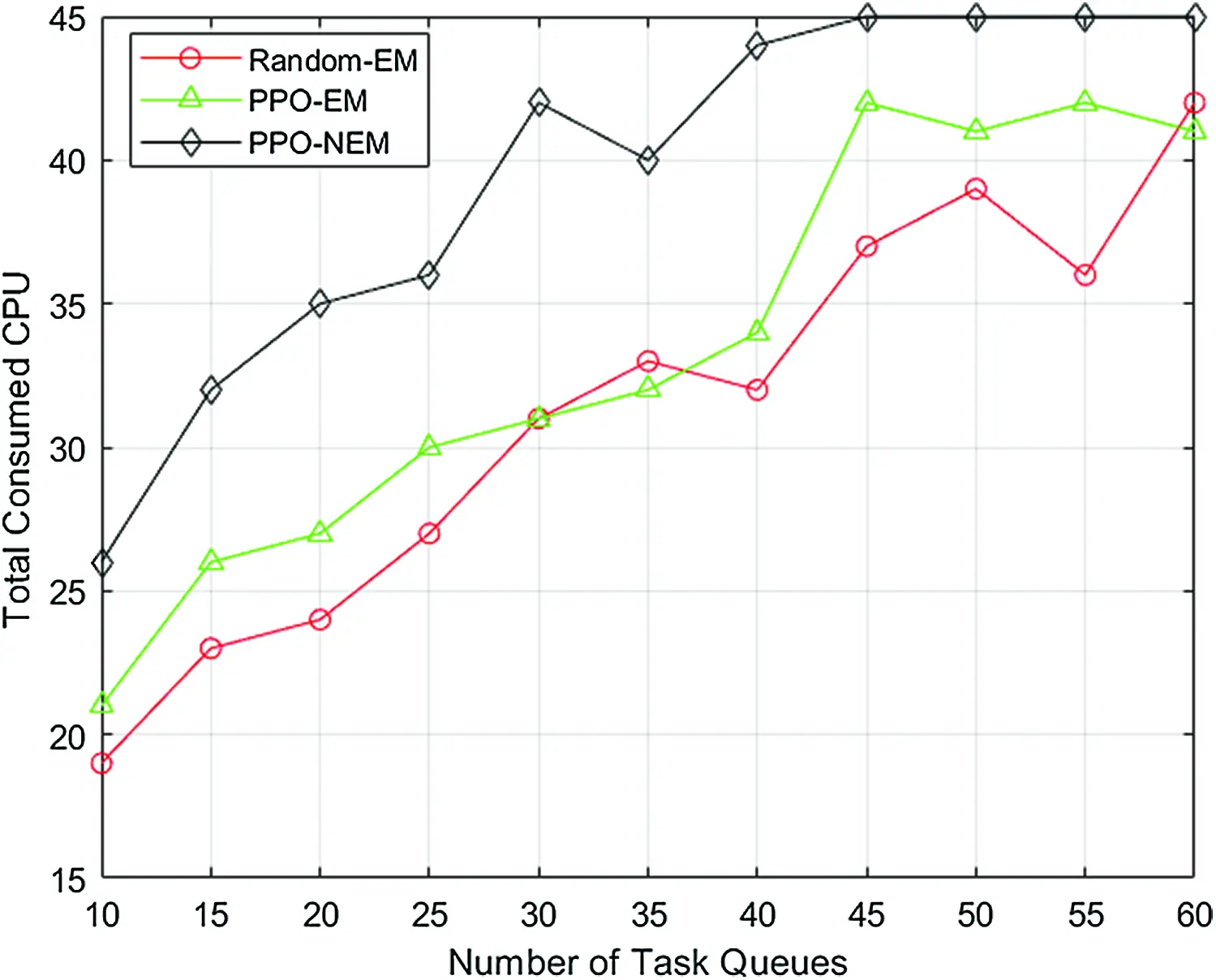[BACK]
 Intelligent Automation & Soft ComputingDOI:10.32604/iasc.2022.018881Article

1Beijing University of Posts and Telecommunications, Beijing, 100876, China
2Communication Operation Center State Grid Henan Electric Power Company, Information & Telecommunication Company, Zheng Zhou, 450052, China
3Fibrlink Communications Co., Ltd, Beijing, 102200, China
4Global Energy Interconnection Research Institute Co., Ltd, Beijing, 102200, China
5Université Paul Sabatier-Toulouse 35, Toulouse, 31000, France
6China Electronics Standardization Institute, Beijing, 100007, China
*Corresponding Author: Meng Chen. Email: 1342029007@qq.com
Received: 24 March 2021; Accepted: 29 April 2021

1  Introduction

In recent years, with the rapid development of Internet of things (IoT) technology, tens of billions of terminal devices (TD) in IoT network have realized economic and efficient interconnection. According to the prediction of Cisco visual network index, by 2023, IoT devices will account for 50% of all networked devices, and the number of connections between devices will reach 14.7 billion . Massive data in IoT network promotes the generation of new services, such as Internet of vehicles, face recognition and so on. These services are delay sensitive tasks and require a lot of computing and storage resources. However, limited by the physical size of the IoT device, the computing and storage resources of terminal devices are limited, and its battery life is short. If tasks are run on the terminal device, it is difficult to meet the user’s requirements, which will lead to bad user experience.In this paper, we first construct a mathematical model including server energy consumption and link energy consumption. Because of the complexity of the edge computing environment, it is necessary to use a reliable and scalable learning algorithm. Based on the above analysis, we design an edge task unloading algorithm based on Proximate Policy Optimization (PPO). The technical contributions of this paper are summarized as follows:

•   To accurately describe the energy consumption of edge network during task unloading, we construct a mathematical model to describe the energy consumption, which includes server energy consumption and link energy consumption. The processing energy consumption of the server is directly proportional to the CPU utilization, and the transmission energy consumption of the link is proportional to the bandwidth utilization.

•   In this paper, the reward function of reinforcement learning algorithm is designed according to the network energy consumption model, the greater the energy consumption, the smaller reward value. Task offload strategy designed in this paper is responsible for selecting the deployable edge server, and the path between adjacent servers is determined by Dijkstra algorithm. Once a task is unloaded, the available resources (environment states) in the edge computing environment are updated.

The rest of this paper is organized as follows: In the second part, this paper briefly reviews related works. In the third part, we construct a mathematical model to describe the energy consumption of edge network, then constructs the problem as ILP model after considering various constraints. In the fifth part, the task unloading algorithm is simulated and the simulation results are analyzed. The last part summarizes the work of this paper and supplements the parts to be improved.

2  Related Work

Dynamic voltage Scaling (DVS) can dynamically adjust voltage frequency to reduce energy consumption and ensure the quality of service of real-time tasks [11,12] studies how to use DVS to unload tasks from terminal devices to edge servers. The experimental results show that DVS can not only complete the task processing within the specified time, but also reduce the terminal energy consumption. [13,14] further discussed the joint optimization of TD energy consumption and task processing time in Mobile Edge Computing (MEC) system using DVS technology, but none of the above studies involved the energy consumption of edge server.

3  Problem Formulation

In this section, we first construct a task unloading model with energy consumption as the optimization goal in IoT. After considering the constraints of bandwidth, computing resources and traffic conservation, we get an optimization problem model.

3.1 Physical Network Model

In this paper, undirected graph G(V,L) is used to represent the edge network, where V is the set of physical nodes in the network, L is the set of physical links in the edge network, lvuL . Computing power of each physical node is represented by Cv , the load capacity of each physical link is represented by Cl , and physical link lvu connects node v and node u .

3.3 Energy Consumption Model

The energy consumption model of edge network constructed in this paper includes the energy consumption of edge server and the energy consumption of physical link transmission traffic.

In addition to the energy consumption of computing tasks, the energy consumption of storage devices and communication devices on the edge server is also considerable. Therefore, the energy consumption of the server is modeled as the power consumption of the server starting up and processing the unloading task. The former is the energy required by the edge server to maintain its normal operation, which depends on whether there are tasks deployed on the edge server, regardless of the number of deployment tasks. The latter is positively correlated with CPU utilization. We use Ntv to indicate the number of tasks t deployed on edge server v .

Ntv=tTzts,tvbtscttvV,tT (1)

Since the energy consumption of the edge server is positively related to the CPU utilization, the processing energy consumption of edge server is calculated as follows:

ptv=phspbsCvcrtNtvtTzts,tvbtscttNtv=phspbsCvcrttTzts,tvbtsctt (2)

pbs is start up energy consumption, and phs is the energy consumption when the edge server is running at full load. Therefore, the energy consumption pv of the edge server can be expressed as:

pv=pbsmin{1,tTtsTSzts,tv}+tTptv (3)

In the above formula, min{1,tTtsTSzts,tv}{0,1} indicates that the power consumption can only be calculated once. tTptv represents the energy consumption generated by all tasks deployed on edge server v .

Similarly, the physical energy consumption in edge network also includes the power consumption of switches on links and the transmission energy consumption when the link transmits the traffic between servers. The former depends on the power on state of the switch on the link, and latter depends on the bandwidth utilization of the physical link. In this section, rl is used to represent the bandwidth utilization of link l . the calculation results are as follows:

rl=tsTSWtsvfug.ytsuvl.btsCllL,tT (4)

wtsvfug indicates that task f on task queue ts is deployed on node v and task g is deployed on node u . ytsuvl indicates whether the task ts deployed on link l passes through node v and node u in turn.

Based on the above analysis, the total energy consumption of l can be calculated as:

pl=pblmin{1,tTtsTSwtsvfugytsuvlbts}+(phlpbl)rl (5)

pbl represents the power consumption of the switch on the link, and min{1,tTtsTSwtsvfugytsuvlbts}{0,1} indicates that the power consumption cannot be calculated repeatedly. Once a task is unloaded on the server, the switch must be kept on. phl represents the energy consumption of the link at full load. Hence, the total energy consumption of the edge network can be expressed as:

ptotal=vVpv+lLpl (6)

3.4 ILP Model

In this section, after considering the constraints of processing sequence of tasks in the queue, computing capacity constraints, and network bandwidth constraints, the unloading problem of terminal tasks is established as an ILP model with energy consumption as the optimization objective.

Firstly, to meet traffic constraints in the process of task unloading, this section assumes that a task sequence to be unloaded is ts , ti and tj are two tasks to be unloaded in ts , and task tj must be executed later. The above constraints are expressed as follows:

C1:uV,gtswtsvfuguV,gtswtsufvg={1iff=ti1iff=tj0otherwise (7)

We assume that the tasks in the task sequence can only be unloaded on one edge server:

C2:vVzts,tv=1 (8)

The tasks in sequences to be unloaded must be unloaded according to the dependency relationship between tasks. The mathematical formula is used to describe the following formula:

C3:uV,vVwtsvfug=xtsfg (9)

In addition, this section also considers the computing capacity constraints of edge servers and the bandwidth constraints of physical links.

C4:NtvcrtCvC5:wtsvfugcttbtsCl (10)

In conclusion, the ILP model for Energy Efficient Task Offload (EETO) problem can be expressed as follows:

minptotal=vVpv+lLplsubject to:C1,C2,C3,C4,C5 (11)

4  Proposed Algorithm

Markov chain is a probability model, the future state is only related to current states. Markov decision process (MDP) is a decision process based on Markov chain. MDP can be represented by a five tuple (S,A,P,R,γ) . S is the state space observed by agent; A represents the action space; P is the set of transition probabilities, the finite set of probabilities that an agent enters a specific state after executing action aiA in a certain state. R represents a set of immediate rewards after performing the action; γ is the discount coefficient.

An edge server in the edge network can be used as an agent to obtain the available resources and task unloading information of the edge network topology through the perceptron installed in physical network. In a certain state, the environment state after agent performs the action is only related to current state, independent of the historical state, and has no aftereffect. Therefore, the edge task unloading problem can be expressed as an MDP model. The problem of edge task offloading based on MDP is presented as follows:

State space S : for slS , and sl=Ucpu(l),Ubw(l) , which indicates the bandwidth resource utilization of CPU and physical link of each edge server in the edge network when l edge task has been unloaded.

Action space A : alA means that the agent selects an edge server in the edge network according to the specific strategy and the current state sl , then deploys the l+1 task in the task queue.

Action execution function: step(sl,a)=rl,sl,l , This function represents the immediate reward rl , subsequent state sl and the number of edge tasks that have been successfully unloaded. If edge task satisfies the constraints (7)–(10) in the process of unloading, it means that the task can be successfully unloaded to the edge network. Reward function Reward(sl,al) represents the immediate reward obtained by the unloading action al in the state sl . The goal of this paper is to reduce the energy consumption in the edge network, therefore, reward function in this paper can be expressed as follows:

Reward(sl,al)=Nptotal (12)

In above formula, the source of action al is from policy π . π is a mapping from state space sl to action space al :

al=π(sl) (13)

The optimization goal of the MDP model established in this paper is to get an optimization strategy, it maximizes the goal of reinforcement learning-the expectation of cumulative return value:

π=argmaxπE[l=0γtReward(sl,al)] (14)

γt is discount factor, and its value decreases with time.

4.2 PPO Algorithm Framework

Because the environment of edge computing network is complex and changeable, to learn in this challenging environment, it is necessary to use a reliable and scalable intelligent algorithm . Because PPO algorithm guarantees stability by binding the range of parameter update to the trust area, this paper considers using this algorithm to complete the unloading of edge tasks .

PPO algorithm is a deep reinforcement learning algorithm based on actor-critic framework. Its architecture contains two actor networks, Actor 1 and Actor 2. Actor 1 represents the latest policy π , which guides the agent to interact with the environment. Critic evaluates the current strategy according to the reward, then updates the parameters in the critic network through back propagation of the loss function. Actor 2 stands for the old strategy πold , after the agent trains a certain number of steps, it uses the parameters in Actor1 to update Actor2. Repeating above process until PPO algorithm converges, we get a trained edge task unloading model based on Actor-Critic framework.

θnew=θold+αθJ (15)

θold and θnew respectively represent the strategy parameters before and after the update, α represents the update step size, and θJ is the objective function gradient. The key of the policy gradient algorithm is the update step size. If the update step size is not selected properly, the algorithm may collapse. PPO decomposes the return function into the return function corresponding to the old strategy plus other items. Once the other items in the new strategy are greater than or 0, the return function can be guaranteed to be monotonous.

η(π~)=η(π)+Es0,a0...π~[t=0γtAπ(st,at)] (16)

Aπ(st,at) is the dominance function. The calculation of the dominance function is shown in the following formula.

Aπ(st,at)=Qπ(s,a)Vπ(s) (17)

PPO algorithm optimizes the parameter θ to satisfy the following equation.

maxθE[πθ(a|s)πθold(a|s)Aθold(s,a)] (18)

where πθ(a|s) is the probability of using strategy π to take action a in state s , and the above formula should satisfy DKLmax(θold,θ)η , DKLmax(θold,θ)η represents the maximum divergence between the old policy parameter and the new policy parameter.

LKLPEN(θ)=Et[πθ(a|s)πθold(a|s)Aθold(s,a)βKL[πθold,π~θ]] (19)

The policy update formula of PPO is shown in the above formula, but there is a problem that the super parameter β is difficult to determine. PPO considers another method to limit the update step size of the policy.

rt(θ)=πθ(at|st)πθold(at|st) (20)

When policy does not change, rt(θ)=1 . and PPO algorithms consider using clip() to limit the similarity between the old and new policies. The improved policy update method is shown in the following formula.

LCLIP(θ)=Et[min(rt(θ)At,clip(rt(θ)),1ε,1+ε)At] (21)

4.3 PPO Algorithm Implement

To optimize the energy consumption in the process of edge task unloading, algorithm designed in this paper mainly includes the following three modules: 1) construction of edge network environment and parameter setting; 2) edge task unloading model training; 3) output of energy consumption aware unloading scheme.

As mentioned in 4.2, the actor network of PPO algorithm designed in this paper is composed of two neural networks, Actor 1 and Actor 2. Actor 1 guides the agent to interact with the environment, obtains transfer samples and caches them. The policy parameters in Actor 2 represents the old policies. After a period of iteration, the parameters in Actor 1 will be used to update the parameters in Actor 2. The critic network consists of a neural network. Training steps of unloading model are as follows:

Step 1: Input current state into Actor 1, and the agent selects an action based on al=π(sl) . After repeating the above process, the agent continuously interacts with the edge network for T time steps, collects the historical interaction information and caches it.

Step 2: Use (17) to calculate the advantage function of each time step.

Step 3: Use the following formula to calculate the loss function of the critic network, update the critic network parameters ϕ according to the function back propagation.

L(ϕ)=t=1T(i>tγitriVϕ(st))2 (22)

Step4: Update the parameters of Actor1 by (17) and (21).

Step5: Repeat step 4. After a certain step, use the network parameters in Actor1 to update the parameters of Actor 2.

Step 6: Repeat steps 1–5.

Based on above analysis, the edge task unloading algorithm based on PPO is described in the following table.5  Performance Evaluation

5.1 Simulation SettingThis paper uses the following four indicators to evaluate the proposed algorithm: 1. Total energy consumption of the network: including energy consumption when processing terminal tasks and energy consumption in the communication process. 2. Number of CPUs: the total number of CPUs consumed when the offload task is executed at the edge network. 3. Physical network bandwidth: the total bandwidth consumed by the physical network when the edge network transmits inter task traffic. 4. Offloading success rate: the percentage of terminal tasks successfully unloaded to the edge server.

To verify the effectiveness of the proposed algorithm. In this section, the algorithm (PPO_EM) proposed in the previous section is compared with random algorithm and task unloading algorithm based on PPO but without considering start up energy consumption (PPO_NEM).

5.2 Simulation Results

PPO_EM is implemented on a computer equipped with inter (R) core i5-9300 h and 16 g memory. The program running environment is Python 3.7.4, Tensorflow 1.15.0; Fig. 2 shows the convergence of PPO_EM in the training process. During training process, the number of task queues to be deployed is set to 80, learning rate of actor network and critical network are set to 0.0001, and reward discount coefficient is set to 0.9. The parameters of Actor 2 are updated with the network parameters in Actor 1 every 15 steps. As can be seen from Fig. 2 at the beginning of training, the results of PPO_EM algorithm fluctuate due to the randomly selected task deployment scheme, but with the increase of training times, reward function gradually converges to the optimal value at about 150 steps.Figure 2: Reward value with the number of iterationsFigure 3: a: Total amount of energy consumption with 5 nodes, 8 physical links b: Total amount of energy consumption with 8 nodes and 12 physical linksFigure 5: Total amount of consumed bandwidthFigure 6: Number of consumed CPUs

6  Conclusion

In this paper, we focus on the optimization of energy consumption of edge server in the process of task unloading. To improve the accuracy of server energy consumption evaluation, we first construct a server energy consumption model including both startup energy consumption and processing energy consumption, then we describe the model as an optimization problem model for energy consumption optimization. Then, a task unloading strategy based on PPO is proposed to solve the approximate optimal task unloading scheme. Simulation results shows that compared with the random algorithm, the proposed algorithm can save 22.69% energy on average.

Funding Statement: This work was supported by State Grid Corporation of China science and technology project “Key technology and application of new multi-mode intelligent network for State Grid” (5700-202024176A-0-0-00).

Conflicts of Interest: We declare that we have no conflicts of interest to report regarding the present study.

## References

1. H. Wang, J. Yong, Q. Liu and A. Yang, “A novel GLS consensus algorithm for alliance chain in edge computing environment,” Computers, Materials & Continua, vol. 65, no. 1, pp. 963–976, 2020.
2. H. Zhang, G. Chen and X. Li, “Resource management in cloud computing with optimal pricing policies,” Computer Systems Science and Engineering, vol. 34, no. 4, pp. 249–254, 2019.
3. Y. Mao, C. You, J. Zhang, K. Huang and K. B. Letaief, “A survey on mobile edge computing: The communication perspective,” IEEE Communications Surveys & Tutorials, vol. 19, no. 4, pp. 2322–2358, 2017.
4. N. R. Sivakumar, “Stabilizing energy consumption in unequal clusters of wireless sensor networks,” Computers, Materials & Continua, vol. 64, no. 1, pp. 81–96, 2020.
5. A. Abdelnasser, E. Hossain and D. I. Kim, “Clustering and resource allocation for dense femtocells in a two-tier cellular OFDMA network,” IEEE Transactions on Wireless Communications, vol. 13, no. 3, pp. 1628–1641, 2014.
6. J. Oueis, E. C. Strinati and S. Barbarossa, “The fog balancing: Load distribution for small cell cloud computing,” in 2015 IEEE 81st Vehicular Technology Conf., Glasgow, UK, pp. 1–6, 2015.
7. J. Rubio, A. Pascual-Iserte, J. del Olmo and J. Vidal, “User association for load balancing in heterogeneous networks powered with energy harvesting sources,” in 2014 IEEE Globecom Workshops, Austin, TX, USA, pp. 1248–1253, 2014.
8. H. M. Wu, Q. S. Wang and K. Wolter, “Tradeoff between performance improvement and energy saving in mobile cloud offloading systems,” in 2013 IEEE Int. Conf. on Communications Workshops, Budapest, Hungary, pp. 728–732, 2013.
9. S. Sardellitti, G. Scutari and S. Barbarossa, “Joint optimization of radio and computational resources for multicell mobile-edge computing,” IEEE Transactions on Signal and Information Processing over Networks, vol. 1, no. 2, pp. 89–103, 2015.
10. L. Chen, S. Zhou and J. Xu, “Computation peer offloading for energy-constrained mobile edge computing in small-cell networks,” IEEE/ACM Transactions on Networking, vol. 26, no. 4, pp. 1619–1632, 2018.
11. J. J. Han and Q. H. Li, “Dynamic power-aware scheduling algorithms for real-time task sets with fault-tolerance in parallel and distributed computing environment,” in 19th IEEE Int. Parallel and Distributed Processing Symp., Denver, CO, USA, pp. 10–14, 2005.
12. Y. Wang, M. Sheng, L. Wang, Y. Zhang, Y. Shi et al., “Energy-optimal partial computation offloading using dynamic voltage scaling,” in 2015 IEEE Int. Conf. on Communication Workshop, London, UK, pp. 2695–2700, 2015.
13. Y. Wang, M. Sheng, X. Wang, L. Wang and J. Li, “Mobile-edge computing: Partial computation offloading using dynamic voltage scaling,” IEEE Transactions on Communications, vol. 64, no. 10, pp. 4268–4282, 2016.
14. Y. Wang, M. Sheng, X. Wang and J. Li, “Cooperative dynamic voltage scaling and radio resource allocation for energy-efficient multiuser mobile edge computing,” in 2018 IEEE Int. Conf. on Communications, Kansas City, MO, USA, pp. 1–6, 2018.
15. N. Yuan, C. Jia, J. Lu, S. Guo, W. Li et al., “A DRL-based container placement scheme with auxiliary tasks,” Computers, Materials & Continua, vol. 64, no. 3, pp. 1657–1671, 2020.
16. L. Li, Y. Wei, L. Zhang and X. Wang, “Efficient virtual resource allocation in mobile edge networks based on machine learning,” Journal of Cyber Security, vol. 2, no. 3, pp. 141–150, 2020.This work is licensed under a Creative Commons Attribution 4.0 International License, which permits unrestricted use, distribution, and reproduction in any medium, provided the original work is properly cited.